# GMAT Math : Graphing an ordered pair

## Example Questions

### Example Question #48 : Graphing

Which quadrants can include the point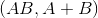if nonzero numbersandhave the same sign?

Explanation:

Ifandhave the same sign, then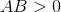, and the point has a positive-coordinate. The sign of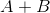is the same as the common sign ofand. Therefore, only the-coordinate restricts the possibilities.

The points witn positive-coordinates are in Quadrant I and Quadrant IV.

### Example Question #49 : Graphing

Which quadrant or axis can include the point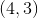?

The-axis

Explanation:

The ordered pairconsists of a positive-coordinate and a positive-coordinate. The only quadrant that consists entirely of positive- and-coordinates is Quadrant I.

### Example Question #50 : Graphing

Which quadrant or axis contains the point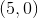?

The-axis

The-axis

The-axis

Explanation:

The ordered pairconsists of a positive-coordinate and a-coordinate of. The only part of the coordinate plane on which all points have a zero-coordinate is the-axis.

### Example Question #1 : Graphing An Ordered Pair

Which of the following ordered pairs is in Quadrant III?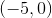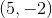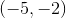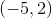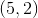Explanation:

By definition, only Quadrant III contains ordered pairs that have both negative- and-coordinates.

### Example Question #2 : Graphing An Ordered Pair

Which quadrant or axis includes the point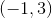?

The-axis

Explanation:

The ordered pairhas a negative-coordinate and a positive-coordinate. By definition, all ordered pairs with negative-coordinates and a positive-coordinates exist in Quadrant II.

### Example Question #3 : Graphing An Ordered Pair

Which quadrant or axis includes the point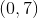?

The-axis

The-axis

The-axis

Explanation:

The ordered pairhas a zero-coordinate and a nonzero-coordinate. By definition, all ordered pairs with zero-coordinates and nonzero-coordinates is on the-axis.

### Example Question #4 : Graphing An Ordered Pair

Which of the following ordered pairs is in Quadrant I?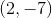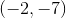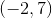None of these coordinates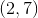By definition, all ordered pairs with positive-coordinates and positive-coordinates are in Quadrant I. Therefore, the only ordered pair in this quadrant is.